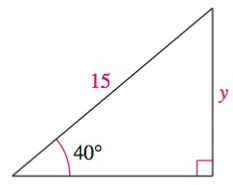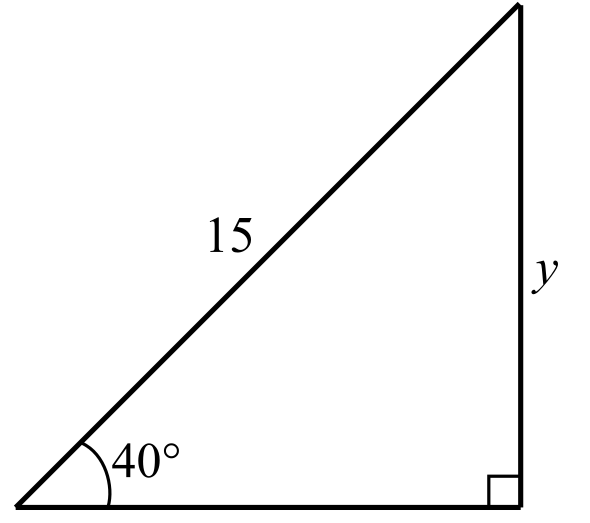Chapter 8.2, Problem 47E### Calculus: An Applied Approach (Min...

10th Edition
Ron Larson
ISBN: 9781305860919

#### Solutions

Chapter
Section### Calculus: An Applied Approach (Min...

10th Edition
Ron Larson
ISBN: 9781305860919
Textbook Problem
1 views

# Solving a Right Triangle In Exercises 43-48, solve for x, y, or r as indicated. SeeSolve for y.To determine

To calculate: The value of y in the provided right angle triangle.Explanation

Given Information:

The provided triangle is shown below:

Formula used:

sin40°0.6427

Right triangle definition:

sinθ=yr         cscθ=rycosθ=xr         secθ=rxtanθ=yx         cotθ=xy

Where, x is the length of the side adjacent to θ, y is the length of the side opposite to θ, and r is the length of hypotenuse.

Calculation:

Consider the given triangle

### Still sussing out bartleby?

Check out a sample textbook solution.

See a sample solution

#### The Solution to Your Study Problems

Bartleby provides explanations to thousands of textbook problems written by our experts, many with advanced degrees!

Get Started

#### Expand each expression in Exercises 122. (x+yxy)2

Finite Mathematics and Applied Calculus (MindTap Course List)

#### In Problems 7-34, perform the indicated operations and simplify. 31.

Mathematical Applications for the Management, Life, and Social Sciences

#### True or False:

Study Guide for Stewart's Multivariable Calculus, 8th